# Binary Lesson 4 Hexadecimal and Binary Practice Modified

• Slides: 17Binary Lesson 4 Hexadecimal and Binary Practice Modified 2 -10 -11 for IPv 6Counting to 15 Base 16 Two Ten (Hex) 0 0 0 1 10 2 2 11 3 3 100 4 4 101 5 5 110 6 6 Base 16 Base Two 1000 1001 1010 1011 1100 1101 1110 Ten 8 9 10 11 12 13 14 (Hex) 8 9 A B C D E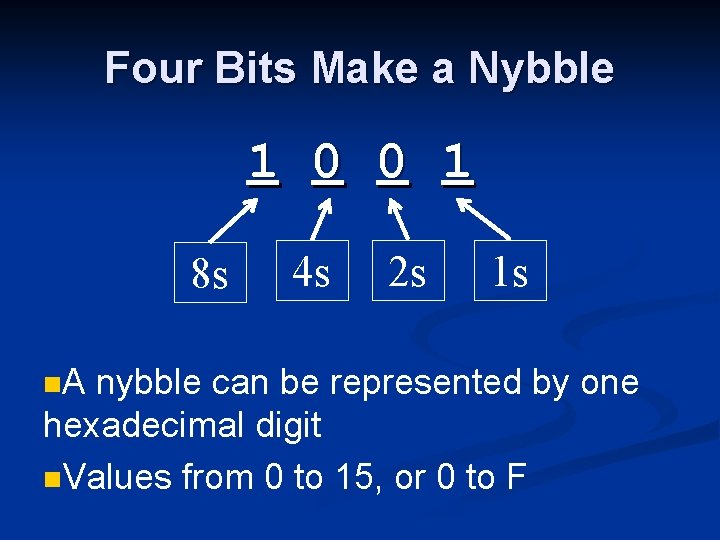Four Bits Make a Nybble 1 0 0 1 8 s n. A 4 s 2 s 1 s nybble can be represented by one hexadecimal digit n. Values from 0 to 15, or 0 to F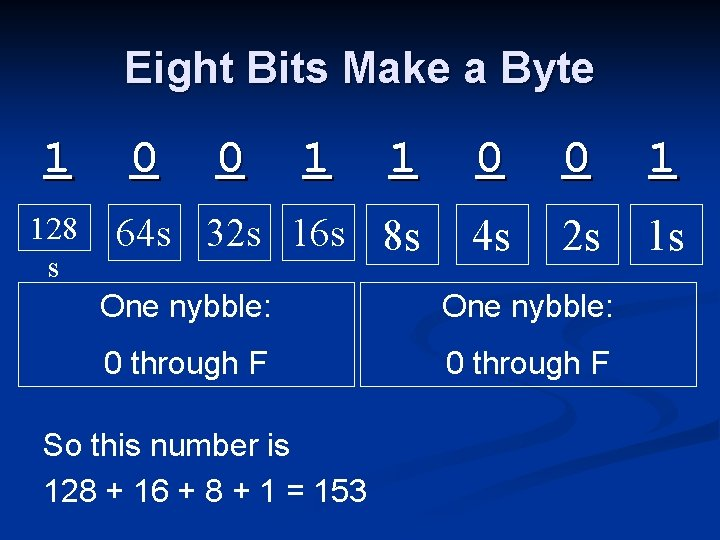Eight Bits Make a Byte 1 128 s 0 0 1 1 0 0 1 64 s 32 s 16 s 8 s 4 s 2 s 1 s One nybble: 0 through F So this number is 128 + 16 + 8 + 1 = 153Two hexadecimal digits make a byte 1 0 0 1 1 0 0 One nybble: 0 through F # of 16 s # of 1 s 1 So this number is \$99 = 9*16 + 9 = 144+9 = 153Binary i. Clicker QuestionsWhat is 16 in hexadecimal? A. B. C. D. E. \$0 A \$A 6 \$0 F \$10 \$16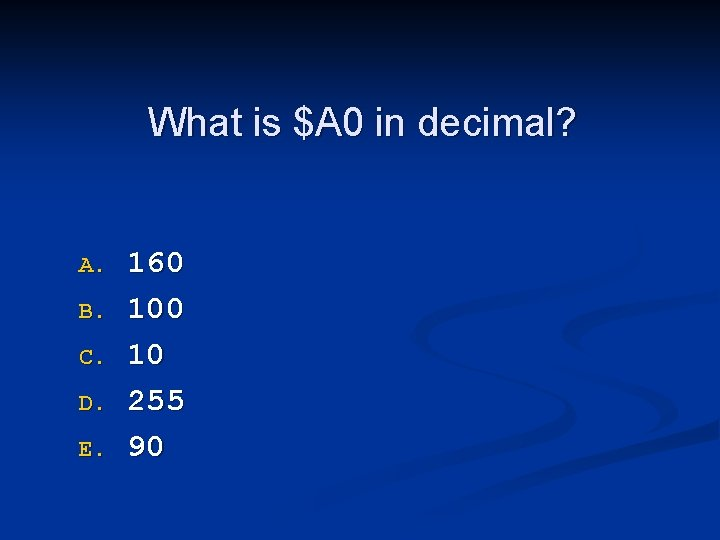What is \$A 0 in decimal? A. B. C. D. E. 160 10 255 90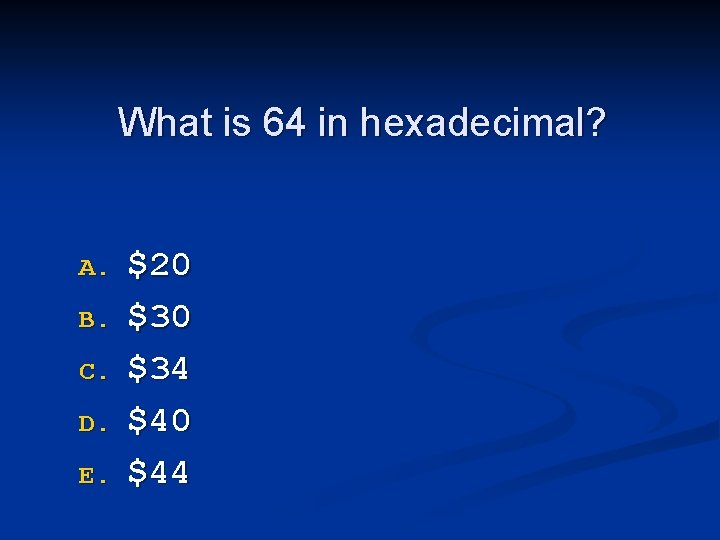What is 64 in hexadecimal? A. B. C. D. E. \$20 \$34 \$40 \$44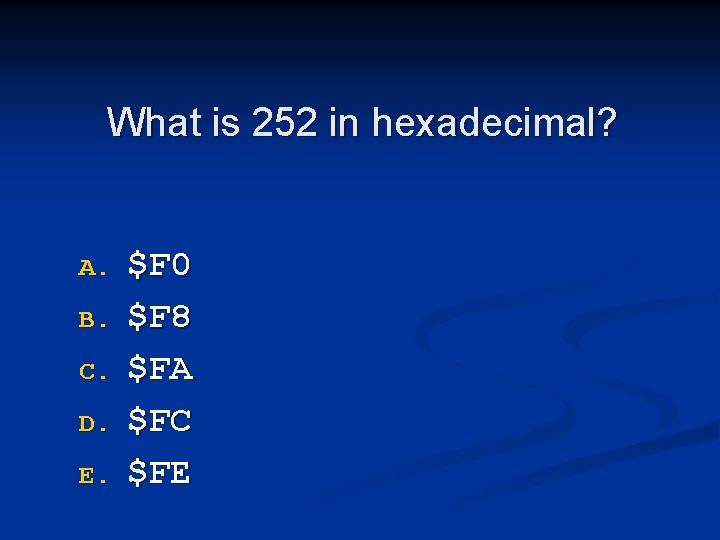What is 252 in hexadecimal? A. B. C. D. E. \$F 0 \$F 8 \$FA \$FC \$FE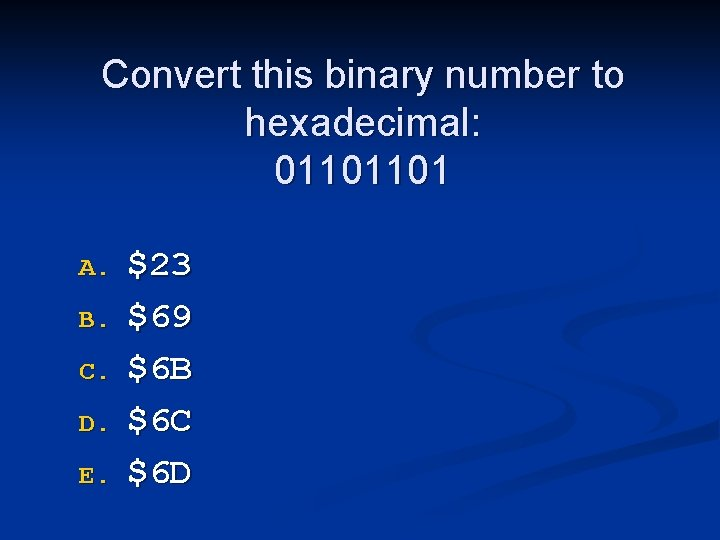Convert this binary number to hexadecimal: 01101101 A. B. C. D. E. \$23 \$69 \$6 B \$6 C \$6 D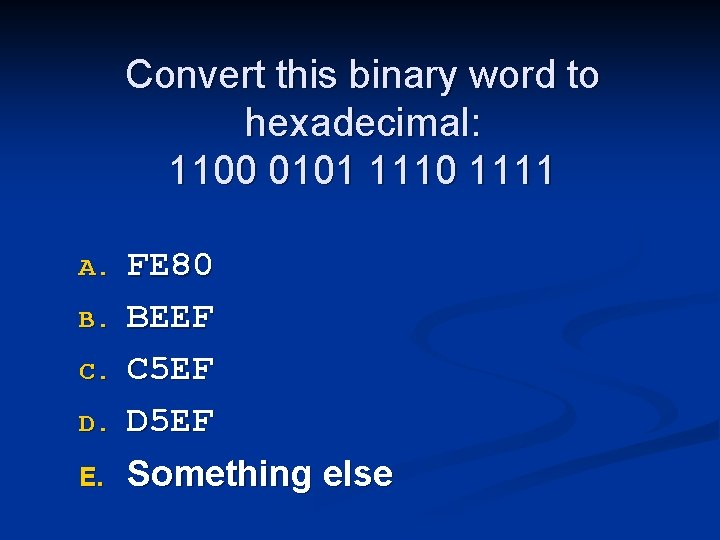Convert this binary word to hexadecimal: 1100 0101 1110 1111 A. B. C. D. E. FE 80 BEEF C 5 EF D 5 EF Something elseConvert this binary word to hexadecimal: 1111 1110 0000 A. B. C. D. E. FE 80 FF 00 FE 00 FD 00 Something elseConvert this hexadecimal word to binary: FE 80 A. B. C. D. E. 1111 1000 1111 1110 1000 1111 1100 1111 1110 1000 Something else 0000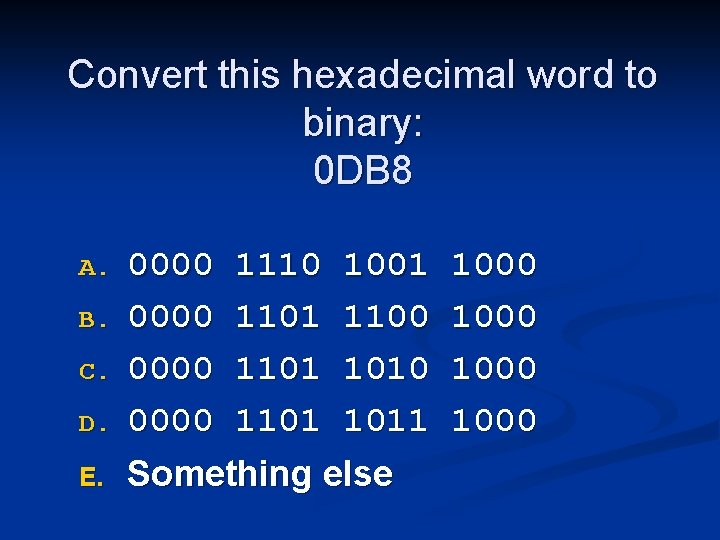Convert this hexadecimal word to binary: 0 DB 8 A. B. C. D. E. 0000 1110 1001 0000 1101 1100 0000 1101 1011 Something else 1000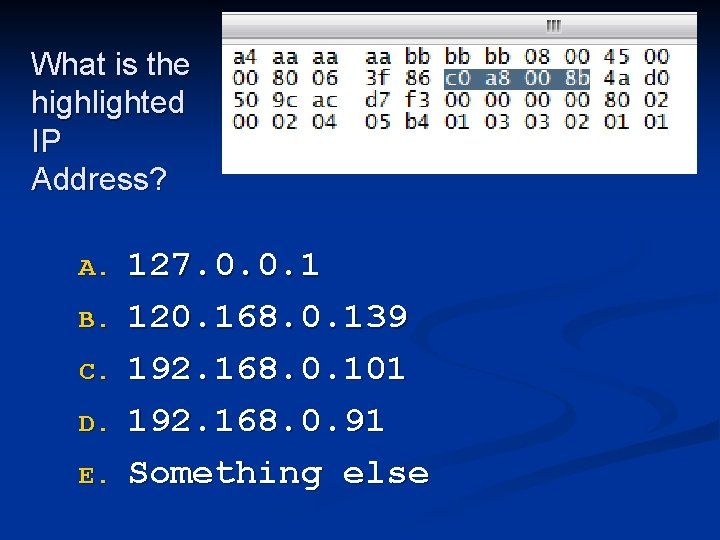What is the highlighted IP Address? A. B. C. D. E. 127. 0. 0. 1 120. 168. 0. 139 192. 168. 0. 101 192. 168. 0. 91 Something else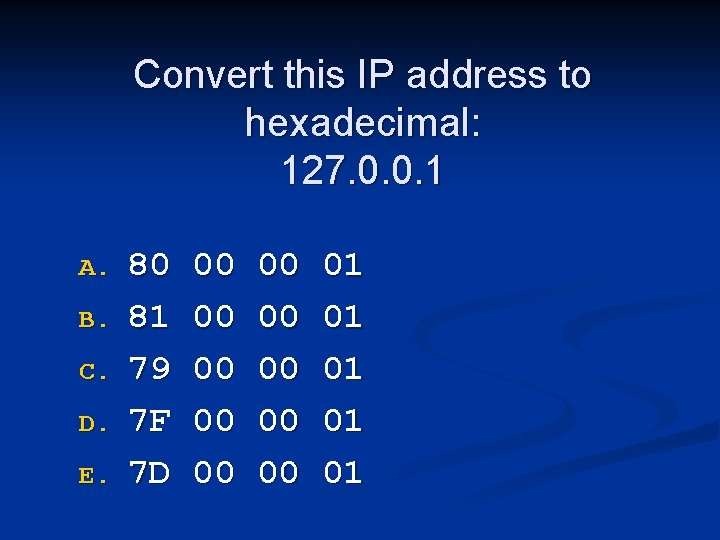Convert this IP address to hexadecimal: 127. 0. 0. 1 A. B. C. D. E. 80 81 79 7 F 7 D 00 00 00 01 01 01